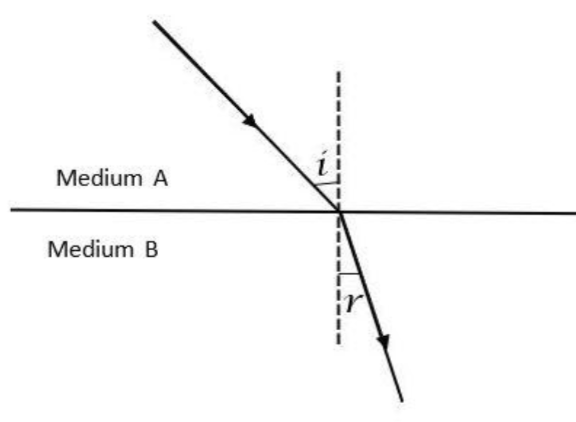QuestionAnswers

# The ratio $\dfrac{\text{sin i}}{\text{sin r}}$ where $\angle i$ and $\angle r$ are the angles of incidence and refraction respectively is called the ______________________ of the second medium (B) with respect to the first medium (A).A). Optical densityB). PowerC). Refractive IndexD). None

Hint: To solve this question, we need to know the basics of refraction. In refraction, the term refracting angle defines the angle that is in the middle of the refracted ray and the normal which is drawn at the point of incidence to the interface where refraction occurs. Keeping this in mind, we can easily solve the question given.

In option C, it is mentioned in the refractive index. We know that the refractive index is the ratio of the speed of light in two different mediums. This refers to the criteria mentioned in the question. It is also the ratio of the sine of the angle of incidence (i) and angle of refraction (r) and is given by $\mu =\dfrac{sini}{sinr}$. this can also be illustrated as follows;